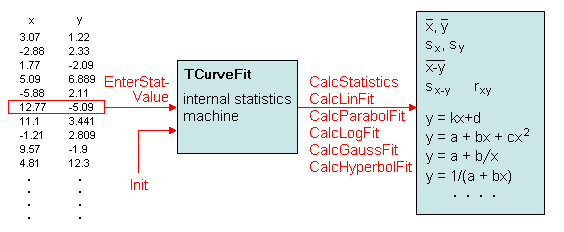The SDL Component Suite is an industry leading collection of components supporting scientific and engineering computing. Please visit the SDL Web site for more information....## TCurveFit

The class TCurveFit provides a simple-to-use means for curve fitting by means of linear regression and splines. In addition, TCurveFit calculates the most important statistical parameters, such as the mean values, the standard deviation, and the correlation coefficient of a series of data pairs. In order to utilize the class TCurveFit, you have to enter the data pairs (x and y) by the method EnterStatValue. The regression parameters can then be obtained by calling the appropriate regression method.The following table summarizes the routines of TCurveFit :

 Init initialize the processing machine EnterStatValue enter a pair of data RemoveStatValue remove a data pair from the pool DataX, DataY direct (read-only) access to the entered data pairs CalcStatistics calculate mean values, standard deviations, and the correlation coefficient CalcAnovaReg performs the analysis of variances for linear models CalcCircleFit calculate the best fitting circle CalcExponentialFit calculate the best exponential fit CalcGaussFit calculate the best Gaussian fit (normal distribution) CalcHoerlFit calculate the best fitting Hoerl function CalcHyperbolFit calculate the best hyperbolic fit to the data CalcLinFit calculate the best linear fit to the data CalcLogFit calculate the best logarithmic fit CalcParabolFit calculate the best parabolic fit to the data CalcPolyFit calculate a best fitting polynomial CalcPowerFit calculate a best fitting power curve CalcReciHyperbolFit calculate the best reciprocal hyperbolic fit to the data CalcReciLinFit calculate the best reciprocal linear fit to the data CalcReciLogFit calculate the best reciprocal logarithmic fit CorrCoeff correlation coefficient CubicSpline calculates a cubic spline interpolation KendallsTau Kendalls's Tau (rank correlation coefficient) KruskalGamma Kruskal's Gamma (rank correlation coefficient) SmoothedSpline calculates a smoothed cubic spline interpolation SpearmanRankCorr calculates Spearman's rank correlation coefficient

In addition, TCurveFit also provides all important univariate parameters for the entered data pairs:

 KurtosisX, KurtosisY kurtosis of x and y values MaxX, MaxY maxima of x and y values MeanDiff mean of differences between x and y MeanAngleX mean of x values interpreted as angles measured in degrees MeanX mean of x values MeanAngleY mean of y values interpreted as angles measured in degrees MeanY mean of y values MinX, MinY minima of x and y values NumData number of data pairs SkewnessX, SkewnessY skewness of x and y values StdDevDiff standard deviation of differences between x and y StdDevX standard deviation of x values StdDevY standard deviation of y values

Last Update: 2012-Oct-20